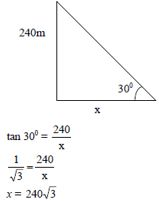# Sample Aptitude Questions of Coal India

1. Type A, 12 kg of rice worth Rs. 40/kg is mixed with Type B, rice worth Rs. 24/kg. What should be the quantity of Type B rice, if the mixture is sold at Rs. 45/kg with 25% profit added in it?
1. 18
2. 48
3. 4
4. Cant Say
C.P. of the mixture = 45/1.25 = Rs. 36/kg..
So, (40-36)/(36-24) = (N)/(12) ⇒ N = 4 Kg.
1. A person spends 1/7th of his salary on travel,1/3rd of the remaining on food, he then spends 1/4th of the remaining on rent. Finally he puts 1/6th of the remaining as a monthly savings, after which he has 25000 left. What is his salary (in Rs.)?
1. 70 K
2. 14 K
3. 84 K
4. 26 K
Let M be the total salary
Therefore, as per question, M × (6/7) × (2/3) × (3/4) × (5/6) = Rs 25000
M = Rs 70000
2. Point C(x,y) divides the distance AB with point A(8,12) and point B(16, 18) in a ratio of 3:5, with AC being shorter than BC. What are the co-ordinates of C?
1. (12,15)
2. (14.5, 12.5)
3. (11,14.25)
4. (8.3, 45)
x co-ordinate of C = [(5×8) + (3×16)] / (3+5) = 11
y co-ordinate of C = [(5×12) + (3×18)] / (3+5) = 14.25
Co-ordinates of C is ( 11, 14.25 )
3. How many terms of the sequence -12, -8, -4,…so on, to make a sum of 120?
1. 11
2. 12
3. 10
4. 13
The series is in A.P. where a = -12 and d = 4.
So 120 = (n/2)[2 × (-12) + (n -1) × 4].
On solving this, we get n = 12
4. A TV set listed at Rs 3200 is sold to a retailer at a successive discount of 25% and 15%. The retailer desires a profit of 20%, after allowing a discount of 10% to the customer. At what price should he list the TV set (in Rs.)?
1. 2720
2. 2448
3. 2448
4. 2133
The retailers C.P. = 3200 × 0.75 × 0.85 = Rs 2040.
His expected S.P. = 2040 × 1.2 = Rs 2448.
But S.P. is 90% of the L.P., as there is a discount of 10%.
So L.P. = 2448/0.9 = Rs 2720
5. What is the average of the first 100 odd natural numbers?
1. 100.5
2. 125
3. 50
4. 100
As we know, sum of first n odd numbers is n2
Therefore, sum of 1st 100 odd numbers is 1002 and average will be 1002/100 = 100.
6. A, B and C invested capitals in the ratio 2 : 3 : 5, the timing of their investment being in the ratio 9 : 5 : 6. The profit if any will be distributed in the ratio
1. 1:2:3
2. 11:8:11
3. 7:2;1
4. 6:5:10
Ratio of capitals = 2 : 3 : 5 and that of time = 9 :5: 6
Therefore, ratio of profits = 2 × 9 : 3 × 5 : 5 × 6 = 18 : 15 : 30 or 6 : 5 : 10
7. It takes 5 sec. for a clock to strike at 5’o clock. If the striking intervals are uniform how much time will it take to strike 9’o clock (in sec.)?
1. 9
2. 10
3. 11
4. 12
There are 4 intervals in 5 strokes.
Time taken to strike 1 stroke will be 5/4 sec.
At 9, there will be 9 strokes and 8 intervals between two strokes.
Thus time required = 5/4 × 8 = 10 sec
8. From the top of the Tower which is 240m high, if the angle of depression of a point on the ground is 30°, then the distance of the point from the foot of the Tower is <
1. 40√3
2. 80√3
3. 120√3
4. 240√3